### Home > A2C > Chapter 12 > Lesson 12.3.2 > Problem12-150

12-150.
1. Let p = 2 + 5i and q = 3 − 4i. Calculate each of the following and give your answer in the form a + bi. Homework Help ✎

1. p − q

2. p · q

3.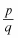4. p2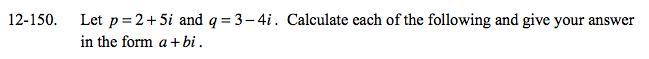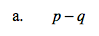(2 + 5i) − (3 − 4i)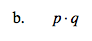Use a generic rectangle.

26 + 7i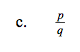Multiply by the conjugate and simplify.

$-\frac{14}{25}+\frac{23}{25}i$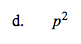See part (b).